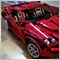# Consecutive Candle EA Coding Assistance16

Hello All,

I could use some assistance with some code I have written.

Basically I am trying to calculate consecutive days of rise or fall.

The issue I am running into is the count isn't correct, it will start off at 0, now lets say its a bullish day it will increment by 1, the next day stays within range of the previous day which resets the count to 0, on the 3rd day its another bullish candle this time rather than increment by 1 the count will show 2,  and for the life of me I am unable to figure out where my logic is wrong.

```int eod=00,dayRF,HighCount,LowCount;
double oneDayH,oneDayL,oneDayC,twoDayH,twoDayL;

void OnTick()
{

Comment(
"The High Count is: ",HighCount,"\n",
"The Low Count is: ",LowCount,"\n",
"The DayRF is: ",dayRF);

oneDayH=iHigh(_Symbol,PERIOD_D1,1);
oneDayL=iLow(_Symbol,PERIOD_D1,1);
oneDayC=iClose(_Symbol,PERIOD_D1,1);
twoDayH=iHigh(_Symbol,PERIOD_D1,2);
twoDayL=iLow(_Symbol,PERIOD_D1,2);

if(Hour()==eod && Minute()==eod && Seconds()==eod)
{
if (oneDayH > twoDayH )
{
LowCount = 0;
HighCount++;
Print("The Consecutive Rise: ",HighCount);
} else HighCount = 0;

if (oneDayL < twoDayL)
{
HighCount = 0;
LowCount--;
Print("The Consecutive Fall: ",LowCount);
} else LowCount = 0;

if (oneDayH < twoDayH && oneDayL > twoDayL)
{
LowCount = 0;
HighCount = 0;
}
else Print("Error Gathering Consecutive Days");

}

if (HighCount > 0) dayRF=HighCount;
else if (LowCount <  0) dayRF=LowCount;
else if (HighCount == 0 && LowCount == 0) dayRF=0;
else Print("Error Displaying Consecutive Days");

}```1328

You will have a result only at 00 h 00 Mn 00 sec ??409

Joe Ramirez:

Hello All,

I could use some assistance with some code I have written.

Basically I am trying to calculate consecutive days of rise or fall.

The issue I am running into is the count isn't correct, it will start off at 0, now lets say its a bullish day it will increment by 1, the next day stays within range of the previous day which resets the count to 0, on the 3rd day its another bullish candle this time rather than increment by 1 the count will show 2,  and for the life of me I am unable to figure out where my logic is wrong.

Your checks to determine the start-of-day is not right. Here's one way:

```int eod=00,dayRF,HighCount,LowCount;
double oneDayH,oneDayL,oneDayC,twoDayH,twoDayL;
bool FirstTick = true;

void OnTick()
{

Comment(
"The High Count is: ",HighCount,"\n",
"The Low Count is: ",LowCount,"\n",
"The DayRF is: ",dayRF);

if (iTime(_Symbol,PERIOD_D1,0)==iTime(_Symbol,PERIOD_M1,0) && FirstTick)
{
FirstTick = false;

oneDayH=iHigh(_Symbol,PERIOD_D1,1);
oneDayL=iLow(_Symbol,PERIOD_D1,1);
oneDayC=iClose(_Symbol,PERIOD_D1,1);
twoDayH=iHigh(_Symbol,PERIOD_D1,2);
twoDayL=iLow(_Symbol,PERIOD_D1,2);

if(Hour()==eod && Minute()==eod && Seconds()==eod)
{
if (oneDayH > twoDayH )
{
LowCount = 0;
HighCount++;
Print("The Consecutive Rise: ",HighCount);
} else HighCount = 0;

if (oneDayL < twoDayL)
{
HighCount = 0;
LowCount--;
Print("The Consecutive Fall: ",LowCount);
} else LowCount = 0;

if (oneDayH < twoDayH && oneDayL > twoDayL)
{
LowCount = 0;
HighCount = 0;
}
else Print("Error Gathering Consecutive Days");

}

if (HighCount > 0) dayRF=HighCount;
else if (LowCount <  0) dayRF=LowCount;
else if (HighCount == 0 && LowCount == 0) dayRF=0;
else Print("Error Displaying Consecutive Days");
}
else
if (iTime(_Symbol,PERIOD_D1,0)!=iTime(_Symbol,PERIOD_M1,0))
FirstTick = true;

}```

After you get the time-check right, then proceed to tweak your comparison of highs and lows (off-hand, you missed one scenario - when the latest candle is both higher and lower than the previous one).16

ffoorr:
You will have a result only at 00 h 00 Mn 00 sec ??
Yes, If I just ran the if statement on tick it would increment by 1 for every tick, the only way I could think of to get the increment by 1 per day would be to run it at 00:00:00.16

Seng Joo Thio:

Your checks to determine the start-of-day is not right. Here's one way:

After you get the time-check right, then proceed to tweak your comparison of highs and lows (off-hand, you missed one scenario - when the latest candle is both higher and lower than the previous one).

Thank you sir for your feedback, I will modify what I have and provide an update.

Udpdate: the updated coded is working like intended for the count to be correct.

Not sure what made my logic incorrect, but I'm glad I reached out, thanks again.

To add comments, please log in or register